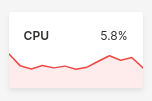# Data Buffers

Data buffers are in-memory data structures designed to hold a card's tabular data.

Data buffers make it convenient to separate data (what is displayed) from presentation (how it is displayed). You can declare a card once, and update its underlying data buffer multiple times. A card can hold zero or more data buffers.

## Cards and buffers​

Data buffers are tabular data structures containing columns and rows. Different cards utilize data buffers in different ways. For example, the plot in the ui.small_series_stat_card() uses a data buffer called `plot_data` to hold time series data.``card = ui.small_series_stat_card(    box=f'1 1 1 1',    title='CPU',    value='10%',    plot_data=data('time usage', -15),    plot_category='time',    plot_value='usage',))``

In the above snippet, `data('time usage', -15)` defines a placeholder for a table with two columns (`time` and `usage`) and 15 rows (we'll get to why the `15` is negative shortly), and the card's `plot_category` and `plot_value` point to the two columns `time` and `usage`, respectively.

Appending rows (tuples or records) to the data buffer make the card plot those rows.

``while True:    card.plot_data[-1] = [get_time(), get_usage()]    time.sleep(1)``

The `while` loop above does something like this:

``card.plot_data[-1] = ['2020-10-05T02:10:20Z', 42.5]card.plot_data[-1] = ['2020-10-05T02:10:21Z', 43.1]card.plot_data[-1] = ['2020-10-05T02:10:22Z', 39.2]card.plot_data[-1] = ['2020-10-05T02:10:23Z', 38.7]``

## Types of buffers​

There are three types of data buffers:

• Array buffers hold tabular data with a fixed number of rows, and allow random access to rows using 0-based integers as keys.
• Cyclic buffers also hold tabular data with a fixed number of rows, but do not allow random access to rows. They can only be appended to. Rows are first-in first-out (FIFO), and adding rows beyond its capacity overwrites the oldest rows.
• Map buffers (or dictionary buffers) hold tabular data with a variable number of rows, and allow random access to rows using string keys.
caution

Map buffers are convenient to use, but use more memory on the server compared to array buffers and cyclic buffers, so use them sparingly.

## The data function​

Use the `data()` function to declare a data buffer. The Wave server uses this declaration to allocate memory for the data buffer.

`data()` takes multiple arguments:

• `fields`: The names of columns, in order; a space-separated string or a list or a tuple (`'foo bar'` or `['foo', 'bar']` or `('foo', 'bar')`.
• `size`: The number of rows to allocate.
• A positive row count creates an array buffer.
• A negative row count creates a cyclic buffer.
• A zero row count (or `None`) creates a map buffer.
• `rows`: A `dict` or `list` of rows to initialize the data buffer with. Each row can be a list or tuple.
• For array or cyclic buffers, pass a list of rows.
• For map buffers, pass a dict with strings as keys and rows as values.
• `columns`: A `list` of columns to initialize the data buffer with. All columns must be of the same length. The columns are automatically transposed to `rows`.
• `pack`: `True` to pack (compress) the provided rows or columns, use less memory on the server-side, and improve performance. Set `pack=True` if you intend to never make any changes to the data buffer once created. Defaults to `False`.

## Buffers in practice​

Declare a 5-row buffer with columns `donut` and `price`.

``# Array bufferb = data(fields='donut price', size=5)# Cyclic bufferb = data(fields='donut price', size=-5)# Map bufferb = data(fields='donut price')``

Declare and initialize a 5-row buffer with columns `donut` and `price`.

``# Array bufferb = data(fields='donut price', size=5, rows=[    ['cream', 3.99],    ['custard', 2.99],    ['cinnamon', 2.49],    ['sprinkles', 2.49],    ['sugar', 1.99],])# Cyclic bufferb = data(fields='donut price', size=-5, rows=[    ['cream', 3.99],    ['custard', 2.99],    ['cinnamon', 2.49],    ['sprinkles', 2.49],    ['sugar', 1.99],])# Map bufferb = data(fields='donut price', rows=dict(    crm=['cream', 3.99],    cst=['custard', 2.99],    cin=['cinnamon', 2.49],    spr=['sprinkles', 2.49],    sug=['sugar', 1.99],))``

Modify a buffer row:

``# Array bufferb = ['cinnamon', 2.99]# Cyclic bufferb[-1] = ['fruit', 2.99] # replaces ['sugar', 1.99]# Map buffer (the following two forms are equivalent)b['cin'] = ['cinnamon', 2.99]b.cin = ['cinnamon', 2.99]``

Modify a buffer value:

``# Array buffer (the following two forms are equivalent)b['price'] = 2.99b.price = 2.99# Cyclic buffer (the following two forms are equivalent)b[-1]['price'] = 2.99 # last donut on menu now costs 2.99b[-1].price = 2.99# Map buffer (the following three forms are equivalent)b['cin']['price'] = 2.99b['cin'].price = 2.99b.cin.price = 2.99``

## Packed buffers​

If you intend to create tabular data once and never change individual rows or values, it is better to avoid allocating memory on the server by using a packed buffer. Packed buffers use less memory on the server and improve performance. To create a packed buffer, use `data(..., pack=True)`. Note that `size` is not required, and is ignored if provided.

``b = data(fields='donut price', rows=[    ['cream', 3.99],    ['custard', 2.99],    ['cinnamon', 2.49],    ['sprinkles', 2.49],    ['sugar', 1.99],], pack=True)``
``b = data(fields='donut price', columns=[    ['cream', 'custard', 'cinnamon', 'sprinkles', 'sugar'],    [3.99 , 2.99, 2.49, 2.49, 1.99],], pack=True)``